# Building a Track for a Carriage to Follow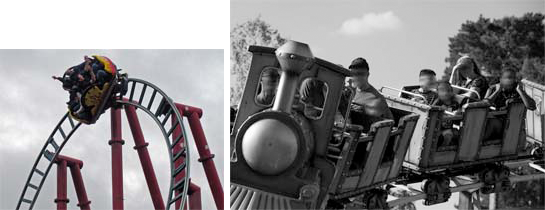In the first image the carriage leans and turns as it follows the track. In the second image the carriage leans and it is the people inside that turn to look around. The 'createTrack' function can be used to simulate either of these two events.

A carriage can be a mesh (with children if wanted), camera or even a point light. A track is a sequence of points in 3D space and for each point rotational data that determines the orientation of the mesh following it, and where necessary any children of the mesh. This rotational data is built by specifying parameters along sections of the track. The default starting position for a carriage is, as you would expect, is with its local x, y and z axis in the directions of the world axes.

A track is created using

var track = createTrack(points, sections);

where points is an array of Vector3s and sections is an array of sections. A section is constructed by giving its starting point (an index of the points array) and an options object (which may be empty), as in

var section0 = new sectionData(0, options0);var section1 = new sectionData(100, {});var section2 = new sectionData(120, options2);var section3 = new sectionData(220, { lean: 0, leanTwists: 0, leanWaves: 0, leanWaveAngle: 0 });var section4 = new sectionData(240, { turn: 0, turnTwists: 0, turnWaves: 0, turnWaveAngle: 0 });

Sections added to the sections array must be in increasing index order. The rotations in any one section are built from the options given in that section and some options from the following section. The following playground allows you to play with these options and others.

PG: Short Track Example

## Section Options

There are two types of rotation 'a lean' and a 'turn' and both may be applied to any section of track. Some illustrative examples are used better understand these. The lean at each point is shown in red and the turn in green, defaults for both are 0. Twists (defaults 0) force complete rotations, waves, with a wave angle, force a rotation to the maximum set by the wave angle followed by a rotation in the reverse direction.

In these examples the track is simply a straight line formed from 100 points and two sections, section0 includes all points from 0 to 99. The second section is an empty section of track but is needed as a device to specify the lean and turn at the track end.

### Leaning

The initial lean comes from section0 and the final lean from section 1.

A lean is the rotation of the carriage about the track direction.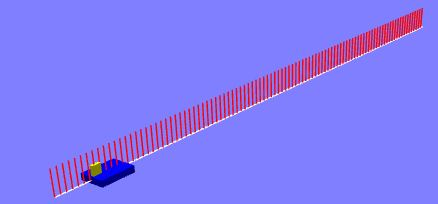initial lean is 0, final lean is zero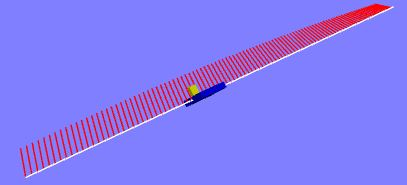initial lean is 0, final lean is π / 2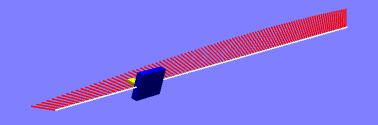initial lean is π / 2, final lean is 0

The value of leanTwists (positive integer, default 0) gives the number of complete rotations about the track over that section of track.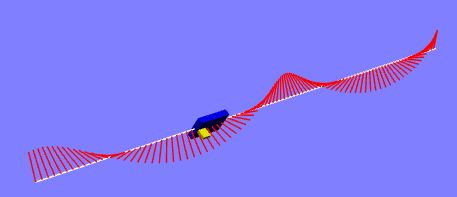initial lean is 0, final lean is 0, leanTwists is 2

The value of waveTwists (positive integer, default 0) gives the number of leans to one side of the track followed by a lean to the other side depending on the lean wave angle over that section of track.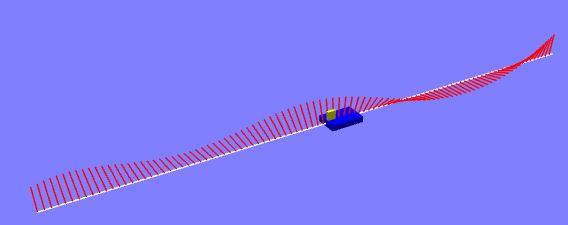initial lean is 0, final lean is 0, leanWaves is 2, leanWaveAngle is π / 2

When both the values of leanWaves and leanWaveAngle or non zero they will override any value given to leanTwists.

### Turning

The initial turn comes from section0 and the final turn from section 1.

A turn is the rotation of the carriage about its local Y axis. (It can alternatively be the rotation of the children of the carriage mesh)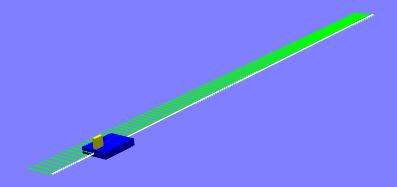initial turn is 0, final turn is zero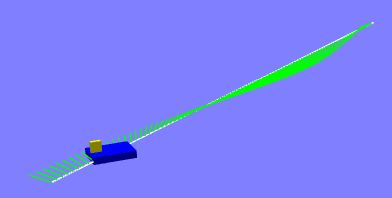initial turn is 0, final turn is π / 2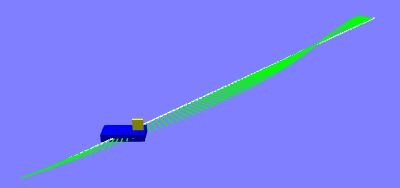initial turn is π / 2, final turn is 0

The value of turnTwists (positive integer, default 0) gives the number of complete rotations about the track over that section of track.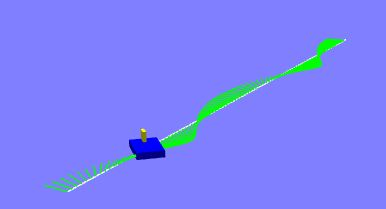initial turn is 0, final turn is 0, turnTwists is 2

The value of waveTwists (positive integer, default 0) gives the number of turns to one side of the track followed by a turn to the other side depending on the turn wave angle over that section of track.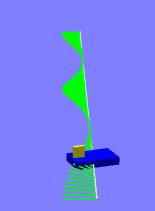initial turn is 0, final turn is 0, turnWaves is 2, turnWaveAngle is π / 2

When both the values of turnWaves and turnWaveAngle or non zero they will override any value given to turnTwists.

### Leaning and Turning

It is possible to use both the lean and turn parameters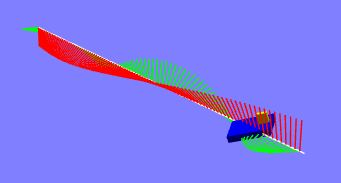both lean and turn are used

### Parameters

The options parameters in a section are

• lean: angle of lean about direction of travel at start of section;
• leanTwists: number of twists against direction of travel over the section;
• leanWaves: number of waves against direction of travel over the section;
• leanWaveAngle: additional angle of lean applied during a wave;
• turn: angle of turn about local carriage y axis at start of section;
• turnTwists: number of twists about local carriage y axis over the section;
• turnWaves: number of waves about local carriage y axis over the section;
• turnWaveAngle: additional angle of turn applied during a wave.

## Data Returned for Track Object

Using

var track = createTrack(points, sections);

gives you a set of arrays of matrices, where each element with index i is the data for the points array at i.

• track.directions: each element is the direction of travel;
• track.rotations: each element is a combination of the lean and turn rotations;
• track.carriageRotations: each element is the lean rotation;
• track.passengerRotations: each element is the turn rotation.

## Closed Tracks

As an example of a closed path take a circle formed from 500 points

var points = [];var n = 400; // number of pointsvar r = 20; //radiusfor (let i = 0; i < n; i++) {  points.push(new BABYLON.Vector3(r * Math.cos((i * 2 * Math.PI) / n), 0, r * Math.sin((i * 2 * Math.PI) / n)));}

When drawing a line to represent the path of the track it is necessary to push points to the end to draw a closed path. However once the line is drawn this must be removed using pop before creating a track.

points.push(points); // push to close pathvar lines = BABYLON.MeshBuilder.CreateLines("lines", { points: points }, scene); //draw path of trackpoints.pop(); // remove before track creation

Also before creating the track the sections needed must be determined. In this case split into four equal sections. Section0 from 0 to 124, section1 from 125 to 249, section2 from 250 to 374 and section 3 from 375 to 0.

var section0 = new sectionData(0, options0);var section1 = new sectionData(125, options1);var section2 = new sectionData(250, options2);var section3 = new sectionData(375, options3);
var sections = [section0, section1, section2, section3];
var track = createTrack(points, sections);

PG: Closed Track Example

## Open Tracks

As an example of a open path take three quarters of a circle formed from 375 points

var points = [];var n = 375; // number of pointsvar r = 20; //radiusfor (let i = 0; i < n; i++) {  points.push(new BABYLON.Vector3(r * Math.cos((i * 3 * Math.PI) / (2 * n)), 0, r * Math.sin((i * 3 * Math.PI) / (2 * n))));}

Drawing a line to represent the path of the track may be done directly.

var lines = BABYLON.MeshBuilder.CreateLines("lines", { points: points }, scene); //draw path of track

Before creating the track the sections needed must be determined. In this case split into three equal sections. Section0 from 0 to 124, section1 from 125 to 249 and section2 from 250 to 374. A fourth dummy section, section3, is needed to provide the data on the final lean and turn at the end of the track. The starting index for the dummy section, at the end, should always be the number of points in the open path, in this case 375.

var section0 = new sectionData(0, options0);var section1 = new sectionData(125, options1);var section2 = new sectionData(250, options2);var section3 = new sectionData(375, options3); //Dummy section
var sections = [section0, section1, section2, section3];
var track = createTrack(points, sections);

PG: Open Track Example

## Using the Built Track

Data from the track can be used to produce quaternions to rotate a mesh or to produce lines showing the direction of rotations or even rails parallel to the track path. Initially just animation around he track is considered.

## From Matrices to Animation

In these examples scene.registerAfterRender is used to create the animation. One aspect that governs the speed of the animation is the distance between the points, so always take this into consideration when designing you path for the track. Of course, depending on how your path is built, you can vary the number of points per length of section. Note that for curved paths it it unlikely a constant speed is obtainable as the distance between points will vary. Often this is not noticeable. You can also produce variations in speed when you construct the quaternions to rotate the carriage and or passengers.

Take a carriage and its contents, forged together by

contents.parent = carriage;

At any point, index i on the track position is from

carriage.position = points[i];

Firstly take the contents as freight, i.e. it moves with the carriage. At any point, index i rotation of both is given by

carriage.rotationQuaternion = BABYLON.Quaternion.FromRotationMatrix(track.rotations[i]);

When you take the contents as a passenger, i.e. somebody who can turn to look around the carriage and passenger rotation are separated

carriage.rotationQuaternion = BABYLON.Quaternion.FromRotationMatrix(track.carriageRotations[i]);contents.rotationQuaternion = BABYLON.Quaternion.FromRotationMatrix(track.passengerRotations[i]);

These can be built from a simple loop.

Now throw a speed variable into the loop. When the speed is 1 or more, an integer s say, then jump from i to i + s. When s is not an integer round it to the nearest integer. When s is less than 1 you need to interpolate between points. When s is 0.25, for example you need 3 extra points between i and i + 1, that is including the point at i 4 points, which is found from 1 / s.

Just considering the x coordinates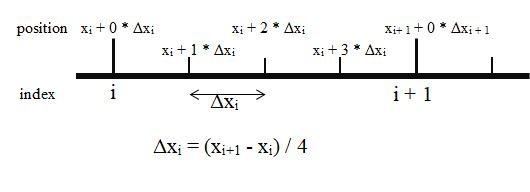between index i and i + 1 the distance to be covered each frame is deltaX = (xi + 1 - xi) / 4 .

The multiplier for deltaX moving along the interpolated points is 0, 1, 2, 3, 0, 1, 2, 3, 0 etc., which is an increasing integer sequence modulo 4.

More generally calling this multiplier the drag on the speed, then drag = Math.round( 1 / speed), to ensure an integer and the multiplier for deltaX is an increasing sequence modulo drag.

Use

var deltaI = 0;
deltaI = (deltaI + 1) % drag;

to obtain the multiplier sequence.

Taking into account speed and whether the contents are freight or passenger you can generate a sequence of positions and rotations from

var nbPoints = points.length;var i = 0;var deltaI = 0;var pace = Math.round(speed);var drag = Math.round(1 / speed);
var carriagePositions = [];var carriageRotations = [];var contentsRotations = [];
var carPosition = BABYLON.Vector3.Zero();
while (i < nbPoints) {  if (speed >= 1) {    carriagePositions.push(points[i]);    if (isFreight) {      carriageRotations.push(BABYLON.Quaternion.FromRotationMatrix(track.rotations[i]));    } else {      carriageRotations.push(BABYLON.Quaternion.FromRotationMatrix(track.carriageRotations[i]));      contentsRotations.push(BABYLON.Quaternion.FromRotationMatrix(track.passengerRotations[i]));    }    i += pace;  } else {    tempPosition.x = points[i].x + (deltaI * (points[(i + 1) % nbPoints].x - points[i].x)) / drag;    tempPosition.y = points[i].y + (deltaI * (points[(i + 1) % nbPoints].y - points[i].y)) / drag;    tempPosition.z = points[i].z + (deltaI * (points[(i + 1) % nbPoints].z - points[i].z)) / drag;
carriagePositions.push(tempPosition.clone());
if (isFreight) {      carriageRotations.push(BABYLON.Quaternion.FromRotationMatrix(track.rotations[i]));    } else {      carriageRotations.push(BABYLON.Quaternion.FromRotationMatrix(track.carriageRotations[i]));      contentsRotations.push(BABYLON.Quaternion.FromRotationMatrix(track.passengerRotations[i]));    }    i = Math.floor(i + (deltaI + 1) / drag);    deltaI = (deltaI + 1) % drag;  }}

### Animation

The animation uses the above in

i = 0;var nbSteps = carriagePositions.length;var animate = true;scene.registerAfterRender(function () {  carrriage.position = carriagePositions[i];  carrriage.rotationQuaternion = carriageRotations[i];  if (!isFreight) {    contents.rotationQuaternion = passengerRotations[i];  }
i += 1;  i %= nbSteps;});

## Showing Axes

The data returned by 'createTrack' can be used to produce the lines showing the intended directions of the local axes 0f the carriage at any of the points (as seen in the images and playgrounds above). These are the required tangents, normals and binormals of the path for the carriage to follow. Initially these are tangent = (1, 0, 0), normal = (0, 1, 0) and binormal = (0, 0, 1).

The following function will produce arrays or tangents, normals and binormals

var tangents = [];var normals = [];var binormals = [];var buildVectors = function() {    normals = [];    binormals = [];    for(let i = 0; i < nbPoints; i++) {        tangents.push(BABYLON.Vector3.TransformNormal(BABYLON.Axis.X, track.railDirections[i]));        if (isFreight) {                normals.push(BABYLON.Vector3.TransformNormal(BABYLON.Axis.Y, track.rotations[i]));                binormals.push(BABYLON.Vector3.TransformNormalToRef(BABYLON.Axis.Z, track.rotations[i]);            }            else {                normals.push(BABYLON.Vector3.TransformNormal(BABYLON.Axis.Y, track.carriageRotations[i]);                binormals.push(BABYLON.Vector3.TransformNormal(BABYLON.Axis.Z, track.carriageRotations[i]);            }        } }

The following function will produce a lineSystem drawing vectors from a series of points and so will draw tangents, normals and binormals.

function drawVectors(vectors, positions, size, color) {  color = color || BABYLON.Color3.White();  size = size || 1;
var lines = [];  for (let i = 0; i < vectors.length - 1; i++) {    var v1 = positions[i];    var v2 = v1.add(vectors[i].scale(size));    lines.push([v1, v2]);  }  var vectorLines = BABYLON.MeshBuilder.CreateLineSystem("vectorLines", { lines: lines }, sc);  vectorLines.color = color;  return vectorLines;}

## Illustrating the Path

### Using a Ribbon to Create a Simple Velodrome

In this next playground a simple velodrome track is built from a ribbon.

PG: Velodrome Example

The normals and binormals of the track are used to create two paths for the ribbon, with a given offset and height. So that the velodrome track sits beneath the wheels a radial vector (from the origin to a point on the track) is used to extend the track outwards.

To produce a smooth velodrome track not all points are necessary. In this example only one in five are used.

for (let i = 0; i < points.length; i += 5) {  radials[i] = points[i].clone().normalize();  upperPoints.push(points[i].add(binormals[i].scale(offset)).add(normals[i].scale(height)).add(radials[i].scale(extend)));  lowerPoints.push(points[i].subtract(binormals[i].scale(offset)).subtract(normals[i].scale(height)).add(radials[i].scale(extend)));}
upperPoints.push(upperPoints); //close pathlowerPoints.push(lowerPoints); //close path
var ribbon = BABYLON.MeshBuilder.CreateRibbon("ribbon", { pathArray: [upperPoints, lowerPoints], sideOrientation: BABYLON.Mesh.DOUBLESIDE }, scene);

Also note that since cylinders are created with their faces horizontal, the wheels are rotated by π / 2 radians and this transformation is baked into the vertices. This ensures that the wheels maintain their intended orientation as the track rotation data is applied.

### Using Tubes and Instances to Create a Roller Coaster

For this roller coaster only the lean angle is used for the carriage and the passenger rotation used to turn the passenger to look out at the start of the run. In this playground paths created either side of the track are used to build tubes and the same track carriage rotational data used to place sleepers underneath the rails.

PG: Roller Coaster Overview PG: Roller Coaster Passenger View

for (let i = 0; i < points.length; i += 5) {  BABYLON.Vector3.TransformNormalToRef(BABYLON.Axis.Y, track.carriageRotations[i], normal);  BABYLON.Vector3.TransformNormalToRef(BABYLON.Axis.Z, track.carriageRotations[i], binormal);  plusPoints.push(points[i].add(binormal.scale(offset)).add(normal.scale(height)));  negPoints.push(points[i].subtract(binormal.scale(offset)).add(normal.scale(height)));
var nsleeper = sleeper.createInstance("");
nsleeper.position.x = points[i].x;  nsleeper.position.y = points[i].y;  nsleeper.position.z = points[i].z;
nsleeper.rotationQuaternion = BABYLON.Quaternion.FromRotationMatrix(track.carriageRotations[i]);  nsleeper.position.subtractInPlace(normal.scale(0.5));}
plusPoints.push(plusPoints);negPoints.push(negPoints);
var plusTube = BABYLON.MeshBuilder.CreateTube("tube", { path: plusPoints, radius: 0.1, tessellation: 4 }, scene);var negTube = BABYLON.MeshBuilder.CreateTube("tube", { path: negPoints, radius: 0.1, tessellation: 4 }, scene);